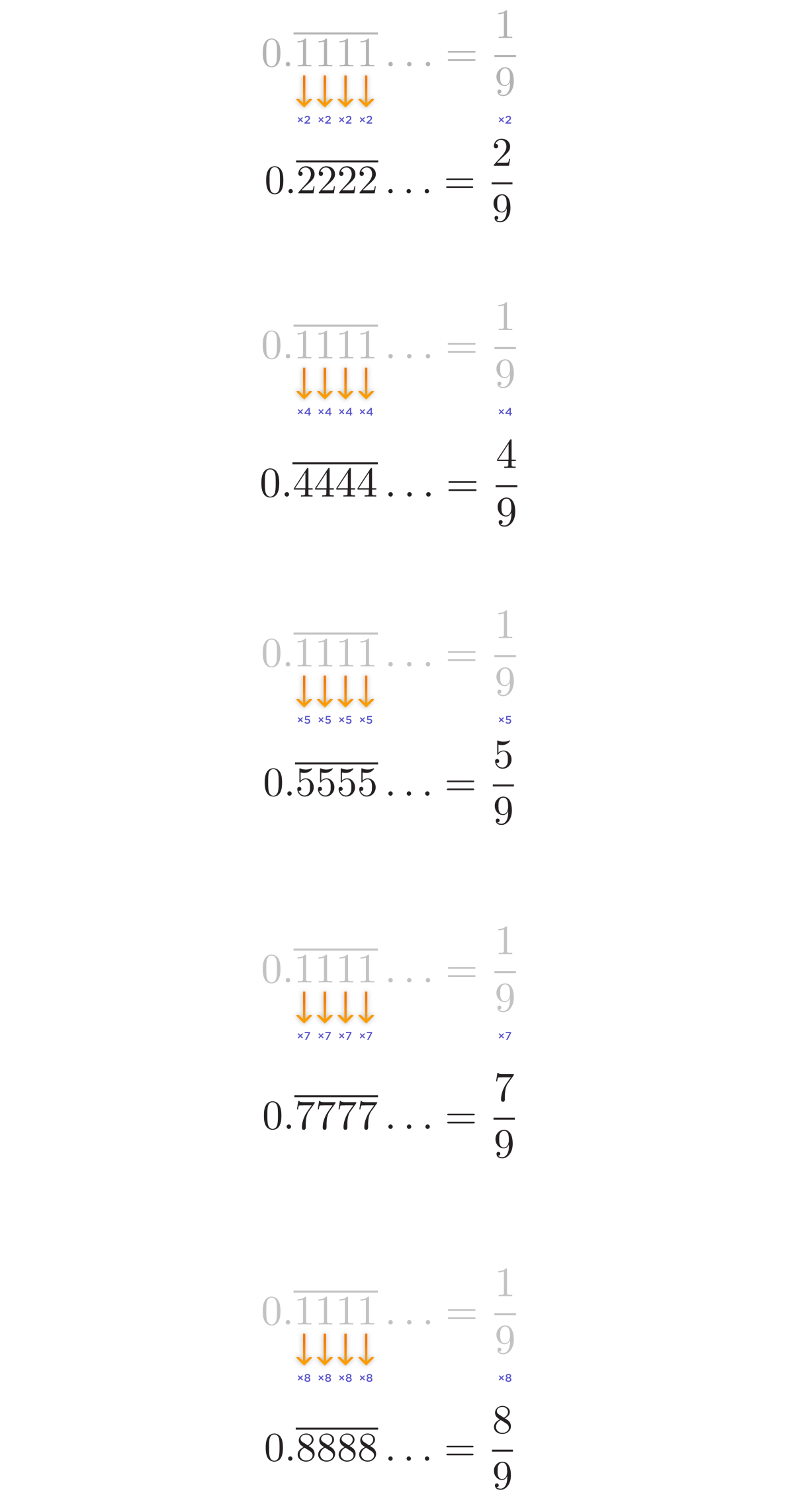# .375 divide by 1000

• .111 divide by 1000, how did it come down 1 over 9. could you please tell me the steps through which you did this

what exercise should one need to do in order to tackle problems like this

hugely thankful

• .111 divide by 1000, how did it come down 1 over 9. could you please tell me the steps through which you did this

what exercise should one need to do in order to tackle problems like this

hugely thankful

I'm going to assume you made a typo and said that 111 divided by 1000, how did it come down to 1 over 9. 111 divided by 1000 is just moving the decimal three spaces to the left, which is going to give you 0.111, which rounds to about 1/9 (0.1 repeated). If you did 0.111 divided by 1000, you'd have 0.000111 which I am just too lazy to convert into a fraction (but it definitely is not 1/9!)

• @funnyorangutan-1 This decimal, $$0.\overline{111}\ldots,$$ is really neat! You can figure out its fraction representation like so:

We know that $$0.\overline{333}\ldots$$ is the same as the fraction $$\frac{1}{3}.$$

If I divide $$0.\overline{333}\ldots$$ by $$3,$$ then each of the digits should turn into a $$1,$$ since the three tenths would turn into a one tenth, the three hundredths would turn into a one hundredth, the three thousandths would turn into a one thousandth, etc.

Dividing the equivalent fraction, $$\frac{1}{3},$$ by $$3,$$ gives us the fraction $$\frac{1}{9}.$$

This will give us the decimal that we want, $$0.\overline{111}\ldots,$$ which is then equal to $$\frac{1}{9}!$$

The same cool pattern applies to the repeating decimal representations of similar fractions whose denominator is $$9,$$ like the ones below:

$$0.\overline{111}\ldots = \frac{1}{9}$$

$$0.\overline{222}\ldots = \frac{2}{9}$$

$$0.\overline{444}\ldots = \frac{4}{9}$$

$$0.\overline{555}\ldots = \frac{5}{9}$$

$$0.\overline{777}\ldots = \frac{7}{9}$$

$$0.\overline{888}\ldots = \frac{8}{9}$$

• could you please further elucidate for the students like myself

i understood this part that we divide .333 by 3 to arrive at 0.111 which turns 3 tenths into 1 tenths and the rest accordingly

but how did we derive 1 / 3 ? did we derive from the fact that 3 divides .333 by .111 so it becomes 1/3

i understood this part - for dividing the 1/3 by 3 is actually multiplying with its reciprocal 1/3 which gives us 1/9

and if i am correct in my above computation then here's my other queries -

For the other numbers - 0.222 if we divide 2 into 0.222 - this would give us .111 same as before, but when we are going to divide 2 by 1/2 which is equivalent of multiplying by reciprocal of 1/2 and it would give us 1/4

How .222 becomes 2/9 or .444 becomes 4/9 and so on?

in my view, every repeating digit such as .333 ; .444 ; .555 can be turned into .111 ( One tenths, One hundredths and so on)

Please do give us your insightful explanation as it motivates student like myself to self explore and form intuitive understanding to these topics.

• @funnyorangutan-1 Thanks for your very polite and curious responses.The first question about why $$\frac{1}{3} = 0.\overline{333}\ldots$$ is quite long, so if you don't mind, I'll put that in it's own post, here. https://forum.poshenloh.com/topic/545/why-is-0-3333Answer to why $$\frac{1}{3} = 0.\overline{333}\ldots$$

For your other question, about why $$0.\overline{222}\ldots = \frac{2}{9} \text{ and } 0.\overline{444}\ldots = \frac{4}{9}$$ and so on, maybe this diagram will help a bit:You can treat $$0.\overline{111}\ldots$$ as a starting point from which you can multiply by any factor in order to get any fraction of the form $$\frac{n}{9},$$ where $$n$$ is an integer.

"Oh, but didn't you leave out the $$\frac{3}{9} \text{ and } \frac{6}{9}?$$"

Very perceptive question!I left those out because $$\frac{3}{9}$$ and $$\frac{6}{9}$$ aren't in simplified form. You wouldn't put "$$\frac{3}{9}$$" on a test as an answer. Instead, you would put $$\frac{1}{3}.$$ And you wouldn't write "$$\frac{6}{9}$$" on a test as an answer either -- you would write $$\frac{2}{3}.$$ So even though the pattern holds for multiplying $$0.\overline{111}\ldots$$ by both $$3$$ and $$6,$$ I left it out, but it is very nice to know that everyone holds across math, and that everything works as it should, because

$$0.\overline{333}\ldots = \frac{3}{9} = \frac{1}{3}$$

Great, math is consistent!When the world seems like it's failing you and there's no end in sight......... there's always math!